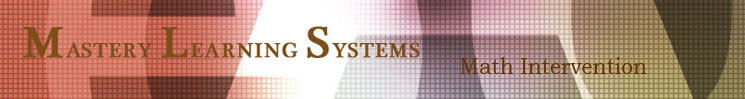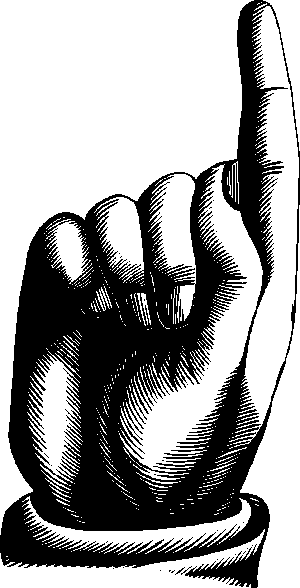K-8 Guided Student Discovery Math for Addition, Subtraction, and Place Value

Emailed to you for School, Homeschool, and Tutor

 Order just the lessons you needin developmentally laid out sections or the whole book with extensive lesson guides, answer keys, and diagnostics. Email orders are placed through PayPal and are processed within 24 hours.Addition, Subtraction, & Place Value Introduction, 18 Complete Chapters (C), The Whole Book
Add, Sub, & Place Value Intro (to get the most out of the lessons in this book)
 Helpful math teaching and learning ideas, learning addition and subtraction, model lesson guides (144 pages) - \$20.19
Place Value to 100
 C - Identify amounts to 10, groups of 10, groups of 10 plus one, amounts from 11 to 20, count in order from 1 to 100 (22 pages) - \$3.19
Plus & Minus 1, 10
 C - Adding and subtracting with number lines, adding 10s (20 pages) - \$2.90
Things That Make 10 & 20
 C - Combinations that make 10 and 20 for fluency in addition and subtraction (38 pages) - \$5.49
Plus & Minus 2, 20
 C - Adding and subtracting with number lines, discovering the relationship between two 10s and 20 (26 pages) - \$3.69
Place Value to 1000
 C - Perceiving and expressing linear amounts on a number line, abstract amounts using money, and standard numerical notation (24 pages) - \$3.39
Plus & Minus 3, 30, 300
 C - Adding and subtracting 3, 30, and 300 to and from numbers up to 1200 with minimal regrouping (26 pages) - \$3.69
Doubles, Regrouping: Carrying 1 Digit
 C - Adding and subtracting double ones ( 6 +/- 6, 6 +/-16), regrouping carrying from the ones digit to the tens (30 pages) - \$4.29
Plus & Minus 4, 40, 400
 C - Adding and subtracting 4, 40, and 400 to and from numbers up to 1200 with minimal regrouping (20 pages) - \$2.90
Regrouping: Exchanging 1 Digit in Subtraction
 C - Exchanging a tens for ten ones, subtracting ones digits with regrouping (16 pages) - \$2.29
Plus & Minus 5, 50, 500
 C - Adding and subtracting 5, 50, and 500 to and from numbers up to 1400 with regrouping (18 pages) - \$2.59
Place Value to 9999
 C - Understanding the place values of 1000s, 100s, 10, and 1s, count, sort, and write numbers to 9999, working with <, =, and >, rounding (20 pages) - \$2.90
Plus & Minus 6, 60, 600, 6000
 C - Adding and subtracting 6, 60, 600, and 6000 to and from numbers up to 9999 with regrouping (from tens to ones only) (18 pages) - \$2.59
Plus & Minus 7, 70, 700, 7000
 C - Adding and subtracting 7, 70, 700, and 7000 to and from numbers up to 9999 with regrouping (from tens to ones only) (18 pages) - \$2.59
Plus & Minus 8, 80, 800, 8000
 C - Adding and subtracting 8, 80, 800, and 8000 to and from numbers up to 9999 with regrouping (from tens to ones only) (18 pages) - \$2.59
Plus & Minus 9, 90, 900, 9000
 C - Adding and subtracting 9, 90, 900, and 9000 to and from numbers up to 9999 with regrouping (from tens to ones only) (24 pages) - \$3.39
Regrouping: Carrying Multiple Digits
 C - Adding multiple digits, regrouping higher digits, including "spillover" carrying (22 pages) - \$3.19
Regrouping: Exchanging Multiple Digits in Subtraction
 C - Subtracting multiple digits, grouping higher digits (14 pages) - \$1.99
Place Value into the Millions
 C - Counting, sorting, & writing numbers to one million, understanding the place values of multiple-digit numbers, rounding, using <, =, > (26 pages) - \$3.69
Place Value of Simple Fractions and Decimals
 C - Counting, sorting, & writing simple fractions and decimals, understanding the place values of simple fractions & decimals, using <, =, > (24 pages) - \$3.39
WB - Addition, Subtraction, and Place Value - \$79.95Watch our Podcasts on how to use the Graphic Pages and Partner Pages to introduce, reinforce,and extend student work in basic Math Concepts and Procedures.
 Mastery Learning Systems, 532 N. School Street, Ukiah, CA 95482, 800-533-4181, mastery@pacific.net